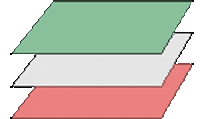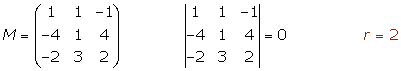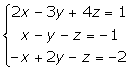To study the intersection of three planes, form a system with the equations of the planes and calculate the ranks.r = rank of the coefficient matrix.

r'= rank of the augmented matrix.

The relationship between three planes presents can be described as follows:

## 1. Intersecting at a Point

r=3, r'=3## 2.1 Each Plane Cuts the Other Two in a Line.

r = 2, r' = 3

The three planes form a prismatic surface.## 2.2 Two Parallel Planes and the Other Cuts Each in a Line

r = 2, r' = 3

Two rows of the coefficient matrix are proportional.## 3.1 Three Planes Intersecting in a Line

r = 2, r' = 2## 3.2 Two Coincident Planes and the Other Intersecting Them in a Line

r = 2, r' = 2

Two rows of the augmented matrix are proportional.## 4.1 Three Parallel Planes

r = 1, r' = 2## 4.2 Two Coincident Planes and the Other Parallel

r = 1, r' = 2

Two rows of the augmented matrix are proportional.## 5. Three Coincident Planes

r = 1, r' = 1State the relationship between the three planes.

1.Each plane cuts the other two in a line and they form a prismatic surface.

2.Each plan intersects at a point.

3.The second and third planes are coincident and the first is cuting them, therefore the three planes intersect in a line.

4.The first and second are coincident and the third is parallel to them.

Did you like the article?(1 votes, average: 5.00 out of 5)Loading...

Emma

I am passionate about travelling and currently live and work in Paris. I like to spend my time reading, gardening, running, learning languages and exploring new places.

Did you like
this resource?

Bravo!